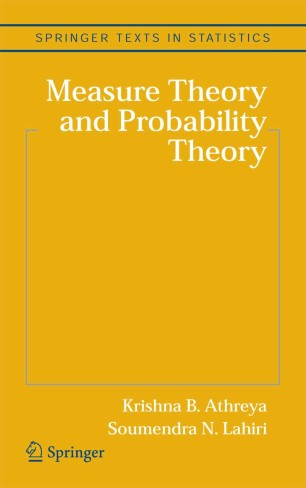# Measure Theory and Probability Theory

• Krishna B. Athreya
• Soumendra N. LahiriTextbook

Part of the Springer Texts in Statistics book series (STS)

1. Front Matter
Pages i-xviii
2. Pages 9-38
3. Pages 39-82
4. Pages 83-111
5. Pages 113-145
6. Pages 147-188
7. Pages 189-218
8. Pages 219-235
9. Pages 237-285
10. Pages 287-315
11. Pages 317-341
12. Pages 343-382
13. Pages 383-398
14. Pages 399-437
15. Pages 439-486
16. Pages 487-507
17. Pages 509-531
18. Pages 533-560
19. Pages 561-571
20. Back Matter
Pages 573-619

### Introduction

This is a graduate level textbook on measure theory and probability theory. The book can be used as a text for a two semester sequence of courses in measure theory and probability theory, with an option to include supplemental material on stochastic processes and special topics. It is intended primarily for first year Ph.D. students in mathematics and statistics although mathematically advanced students from engineering and economics would also find the book useful. Prerequisites are kept to the minimal level of an understanding of basic real analysis concepts such as limits, continuity, differentiability, Riemann integration, and convergence of sequences and series. A review of this material is included in the appendix.

The book starts with an informal introduction that provides some heuristics into the abstract concepts of measure and integration theory, which are then rigorously developed. The first part of the book can be used for a standard real analysis course for both mathematics and statistics Ph.D. students as it provides full coverage of topics such as the construction of Lebesgue-Stieltjes measures on real line and Euclidean spaces, the basic convergence theorems, L^p spaces, signed measures, Radon-Nikodym theorem, Lebesgue's decomposition theorem and the fundamental theorem of Lebesgue integration on R, product spaces and product measures, and Fubini-Tonelli theorems. It also provides an elementary introduction to Banach and Hilbert spaces, convolutions, Fourier series and Fourier and Plancherel transforms. Thus part I would be particularly useful for students in a typical Statistics Ph.D. program if a separate course on real analysis is not a standard requirement.

Part II (chapters 6-13) provides full coverage of standard graduate level probability theory. It starts with Kolmogorov's probability model and Kolmogorov's existence theorem. It then treats thoroughly the laws of large numbers including renewal theory and ergodic theorems with applications and then weak convergence of probability distributions, characteristic functions, the Levy-Cramer continuity theorem and the central limit theorem as well as stable laws. It ends with conditional expectations and conditional probability, and an introduction to the theory of discrete time martingales.

Part III (chapters 14-18) provides a modest coverage of discrete time Markov chains with countable and general state spaces, MCMC, continuous time discrete space jump Markov processes, Brownian motion, mixing sequences, bootstrap methods, and branching processes. It could be used for a topics/seminar course or as an introduction to stochastic processes.

Krishna B. Athreya is a professor at the departments of mathematics and statistics and a Distinguished Professor in the College of Liberal Arts and Sciences at the Iowa State University. He has been a faculty member at University of Wisconsin, Madison; Indian Institute of Science, Bangalore; Cornell University; and has held visiting appointments in Scandinavia and Australia. He is a fellow of the Institute of Mathematical Statistics USA; a fellow of the Indian Academy of Sciences, Bangalore; an elected member of the International Statistical Institute; and serves on the editorial board of several journals in probability and statistics. Soumendra N. Lahiri is a professor at the department of statistics at the Iowa State University. He is a fellow of the Institute of Mathematical Statistics, a fellow of the American Statistical Association, and an elected member of the International Statistical Institute.

### Keywords

Markov Chain Markov Chains Measure Probability distribution Probability space Probability theory Stochastic Processes analysis linear optimization real analysis statistics

#### Authors and affiliations

• Krishna B. Athreya
• 1
• Soumendra N. Lahiri
• 2
1. 1.Department of Mathematics and Department of StatisticsIowa State UniversityAmes
2. 2.Department of StatisticsIowa State UniversityAmes

Industry Sectors
Pharma
Biotechnology